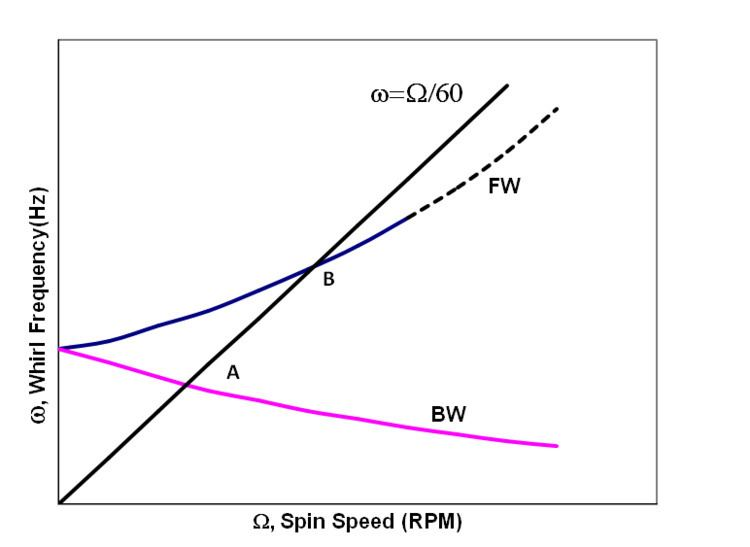# Campbell diagram

Updated onA Campbell diagram plot represents a system's response spectrum as a function of its oscillation regime. It is named for Wilfred Campbell, who introduced the concept., also called interference diagram.

## In rotordynamics

In rotordynamical systems, the eigenfrequencies often depend on the rotation rates due to the induced gyroscopic effects or variable hydrodynamic conditions in fluid bearings. It might represent the following cases:

1. Analytically computed values of eigenfrequencies as a function of the shaft's rotation speed. This case is also called "whirl speed map". Such chart can be used in turbine design as shown in the numerically calculated Campbell diagram example illustrated by the image: analysis shows that there are well-damped critical speed at lower speed range. Another critical speed at mode 4 is observed at 7810 rpm (130 Hz) in dangerous vicinity of nominal shaft speed, but it has 30% damping - enough to safely ignore it.

2. Experimentally measured vibration response spectrum as a function of the shaft's rotation speed (waterfall plot), the peak locations for each slice usually corresponding to the eigenfrequencies.

## In acoustical engineering

In acoustical engineering, the Campbell diagram would represent the pressure spectrum waterfall plot vs the machine's shaft rotation speed (sometimes also called 3D noise map).

Topics